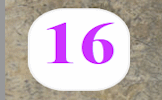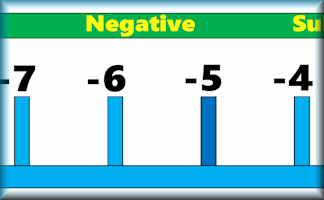# Number LineLeftmost number: showing a

#### Don't Shoot The SquareYou will need to be quick on the draw to shoot all of the numbers except the square numbers. So far this activity has been accessed 57806 times and 473 people have earned a Transum Trophy for completing it.

## Number Line

#### For the teaching of decimal fractions see the Decimals Line.

Change the numbers displayed on the number line by using the dropdown boxes above. The two arrows are available to drag up to the number line to show certain values.

This number line can help you explain directed number arithmetic. For example to show the calculation 6 - 10 you could start by moving the blue arrow to show positive six. Next use the red arrow to move ten units to the left (equivalent to minus ten) ending at minus four.

To show the calculation - 4 - - 7 begin with the blue arrow at minus four. The red arrow can then show that subtracting negative seven equates to a move to the right ending at positive three.

There are many more Negative Numbers activities on Transum.org. There are pupil activities, teacher resources and lesson starters.

If you are a Transum subscriber there is a colourful, printable Number Line banner to print out for your classroom. No Mathematics room should be without a number line on the wall for a quick and easy reference when adding and subtracting.For All:

©1997-2021 WWW.TRANSUM.ORG